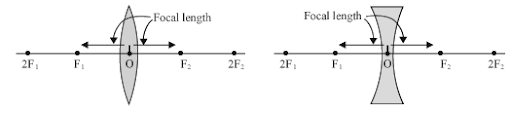# Draw a figure explaining various terms related to a lens

more_vert
Draw a figure explaining various terms related to a lens

more_vert

verified

Various Terms related with Lenses:

1) Optical centre : The point inside a lens on the principal axis, through which light rays pass without changing their path is called the optical centre (O) of the lens.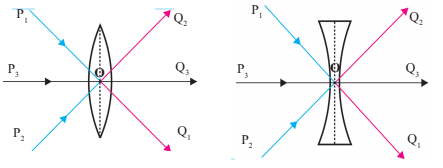2) Centre of curvature : The centres of the spheres whose parts form the surfaces of a lens are called the centres of curvature of the lens. A lens has two centres of curvature C1 and C2 for its two spherical surfaces.3) Radius of curvature : The distance of the optical centre from either of the centre of curvatures is termed as the radius of curvature.

4) Principal axis : The imaginary straight line joining the two centers of curvature and the optical centre (O) is called the principal axis of the lens.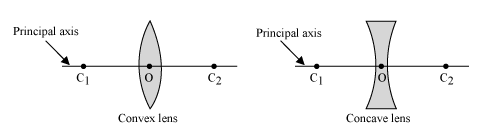5) Focus (Principal focus) (F) : When light rays parallel to the principal axis are incident on a convex lens, they converge at a point on the principal axis. This point is called the principal focus (F) of the convex lens.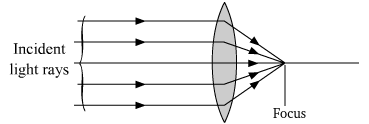Light rays travelling parallel to the principal axis of a concave lens diverge after refraction in such a way that they appear to be coming out of a point on the principal axis. This point is called the principal focus of the concave lens. A lens has two principal foci F1 and F2.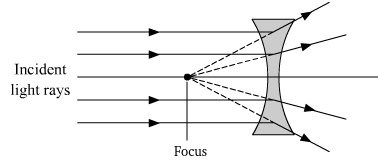6) Focal length : The distance between the focus (F1 or F2) and the optical centre (O) is known as the focal length of the lens.